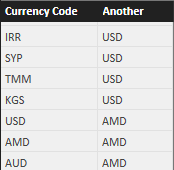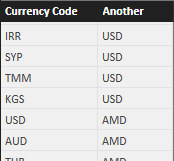The only difference between the two queries is the expression in the “Another” column.

In Q1 you have the following code:

```        "Another", Currency[Currency Code]
```

Whereas, in Q2 you have this different expression:

```        "Another", Currency[Currency Code] & ""
```

In both queries, CROSSJOIN produces the Cartesian product of all the currencies with themselves. Starting from 28 currencies, you obtain 784 rows, as you can see in the table CrossJoinResult of the solution file.The result produced by CROSSJOIN is then filtered by FILTER, which keeps only the rows that have different values in both columns, as you can see in the table FilterResult of the solution file.Up to now, the visible result is identical for Q1 and Q2. However, the internal metadata is different. In fact, the Another column of Q1 keeps the lineage to Currency Code column, whereas in Q2 it is a completely new column, unrelated with the previous one.

When you apply this result as a filter to the original Currency table, the combinations of columns are applied to the original Currency Code column in the Currency table. Also the “Another” column is a reference to the original Currency Code column. The filter argument of CALCULATE is a table of two columns, so the combinations of values in the two columns of the filter have to exists in the result. However, we removed from the table the rows having an identical value in the two columns of the CROSSJOIN result. In other words, the CALCULATE statement for Q1 is trying to do something similar to this:

```FILTER (
Currency,
CONTAINS (
FILTER (
Q1,
Q1[Currency Code] = Currency[Currency Code]
&& Q1[Another] = Currency[Currency Code]
)
)
)
```

Thus, if you do not have rows with identical values in “Currency Code” and “Another” columns, no rows will be filtered from Currency. For this reason, Q1 returns an empty table.

In case of Q2, the data lineage is broken by the expression that concatenates an empty string to the existing currency code. Even if the table looks identical, the missing data lineage produces the following equivalent behavior of CALCULATE in Q2:

```FILTER (
Currency,
CONTAINS (
FILTER (
Q1,
Q1[Currency Code] = Currency[Currency Code]
)
)
)
```

The “Another” column is ignored because it does not apply any filter to the filter context. Remember, the FILTER syntaxes we used in this explanation is just for educational purposes, it does not correspond to the inner execution of CALCULATE.

As a result, Q2 returns all the rows of the Currency table.